# ISEE Lower Level Quantitative : Plane Geometry

## Example Questions

← Previous 1 3 4 5 6 7 8 9 60 61

### Example Question #1 : Quadrilaterals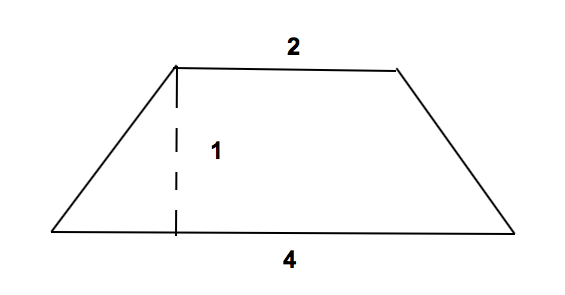What is the area of a trapezoid if its height is 1, its long base is 4, and its short base is 2?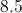Explanation:

The area of a trapezoid is given by the formula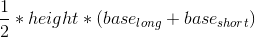.

We know that the height is 1, the long base is 4, and the short base is 2.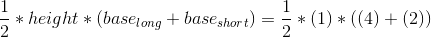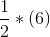### Example Question #1 : How To Find The Area Of A Trapezoid

Which formula would you use to find the area of a trapezoid?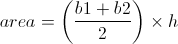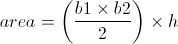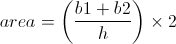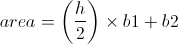Explanation:

The find the area of a trapezoid, use: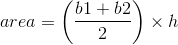### Example Question #1 : Trapezoids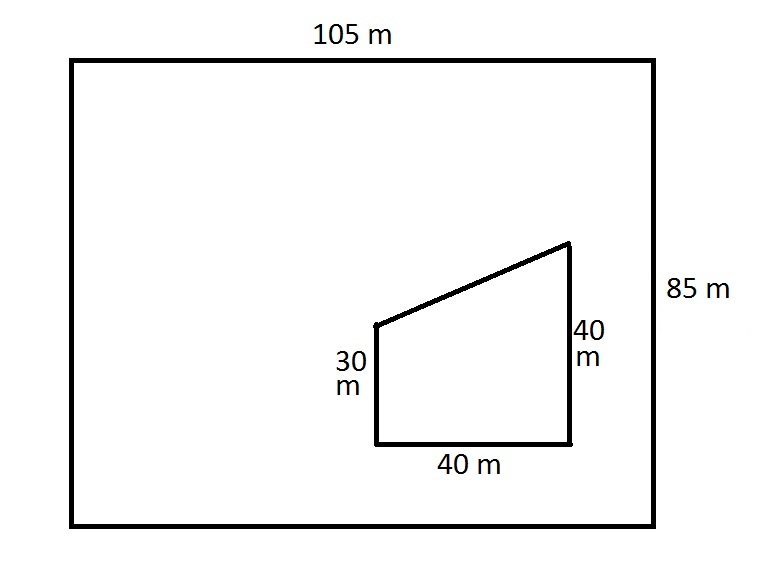The above diagram shows a trapezoidal home within a rectangular yard. What is the area of the yard?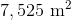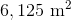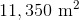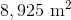Explanation:

The area of the yard is the area of the small trapezoid subtracted from that of the large rectangle. The area of a rectangle is the product of its length and its height, so the large rectangle has area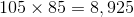square meters.

The area of a trapezoid is half the product of its height and the sum of its two parallel sides (bases), so the small trapezoid has area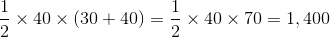square meters.

The area of the yard is the difference of the two: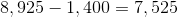square meters.

### Example Question #1 : How To Find The Area Of A Trapezoid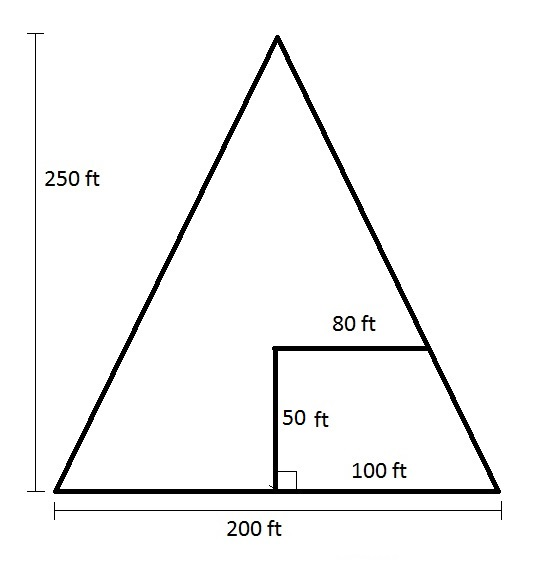Note: Figure NOT drawn to scale.

Mr. Smith owns the triangular piece of land seen in the above diagram. He sells the trapezoidal parcel shown at bottom right to his brother. What is the area of the land he retains?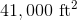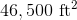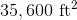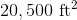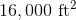Explanation:

The area of a triangle is half the product of its base and its height, so Mr. Smith's parcel originally had area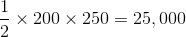square feet.

The area of a trapezoid is the half product of its height and the sum of its two parallel sides (bases), so the portion Mr. Smith sold to his brother has area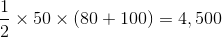square feet.

Therefore, Mr. Smith retains a parcel of area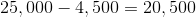square feet.

### Example Question #1 : How To Find The Area Of A Trapezoid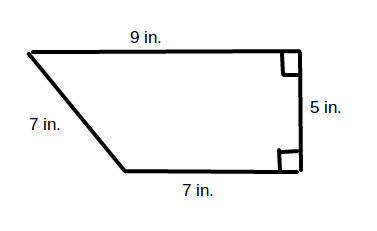What is the area of the above trapezoid?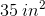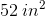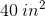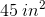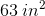Explanation:

The formula for the area of a trapezoid is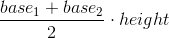In other words, find the average of the bases and multiply by the height. Substituting in the values of the bases for the given trapezoid,and, you get: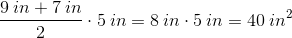### Example Question #231 : Geometry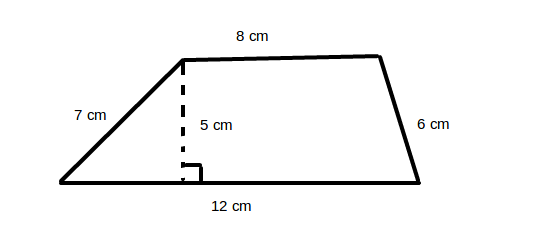What is the area of the above trapezoid?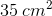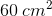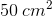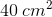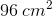Explanation:

The formula for the area of a trapezoid isIn other words, find the average of the bases and multiply by the height. Substituting the values of the bases of the given trapezoid,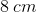and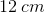, into the equation, you get: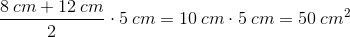The area of the trapezoid is thus.

### Example Question #1 : Trapezoids

Find the perimeter of the following trapezoid.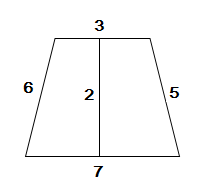Explanation:

In order to find the perimeter of the trapezoid, we have to add up all the outer sides: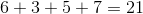### Example Question #1 : Trapezoids

Find the perimeter of the following trapezoid.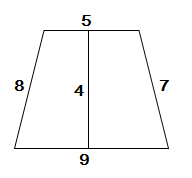Explanation:

In order to find the perimeter we must add up all the outer sides of the trapezoid.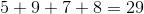### Example Question #2 : Trapezoids

Daria found the perimeter of the below trapezoid to be 29 inches.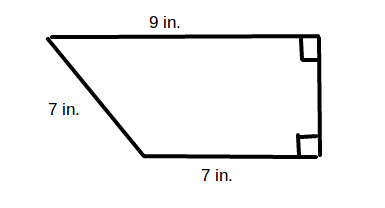What is the length of the missing side?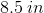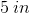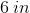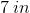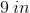Explanation:

To find the perimeter of a trapezoid, add up the lengths of its four sides. In the given trapezoid,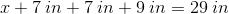To find the missing side, combine like terms and solve for: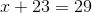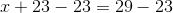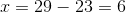The missing side of the trapezoid islong.

### Example Question #1 : Plane GeometryWhat is the perimeter of the above trapezoid?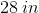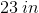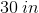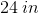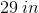Explanation:

To find the perimeter of a trapezoid, add up the lengths of the four sides. In the given trapezoid,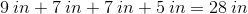← Previous 1 3 4 5 6 7 8 9 60 61

### All ISEE Lower Level Quantitative Resources